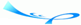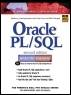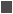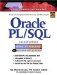# Lab 4.1 Making Use of DML in PLSQL

 Team-FlyOracle® PL/SQL® Interactive Workbook, Second EditionBy Benjamin Rosenzweig, Elena Silvestrova Table of Contents Chapter 4.  SQL in PL/SQL

### Lab 4.1 Making Use of DML in PL/SQL

Lab Objectives

After this Lab, you will be able to:

• Use the SELECT INTO Syntax for Variable Initialization

• Use DML in a PL/SQL Block

• Make Use of a Sequence in a PL/SQL Block

#### Variables Initialization with SELECT INTO

In PL/SQL, there are two main methods of giving value to variables in a PL/SQL block. The first one, which you learned in Chapter 2, "PL/SQL Concepts," is initialization with the ":=" syntax. In this lab we will learn how to initialize a variable with a select statement by making use of SELECT INTO syntax.

A variable that has been declared in the declaration section of the PL/SQL block can later be given a value with a SELECT statement. The correct syntax is as follows:

` SELECT item_name     INTO variable_name     FROM table_name; `

It is important to note that any single row function can be performed on the item to give the variable a calculated value.FOR EXAMPLE

` --  ch04_1a.sql  SET SERVEROUTPUT ON  DECLARE     v_average_cost VARCHAR2(10);  BEGIN     SELECT TO_CHAR(AVG(cost), '\$9,999.99')       INTO v_average_cost       FROM course;     DBMS_OUTPUT.PUT_LINE('The average cost of a '||        'course in the CTA program is '||        v_average_cost);  END; `

In this example, a variable is given the value of the average cost of a course in the course table. First, the variable must be declared in the declaration section of the PL/SQL block. In this example, the variable is given the datatype of VARCHAR2(10) because of the functions used on the data. The same select statement that would produce this outcome in SQL*Plus would be

` SELECT TO_CHAR(AVG(cost), '\$9,999.99')    FROM course; `

The TO_CHAR function is used to format the cost; in doing this, the number datatype is converted to a character datatype. Once the variable has a value, it can be displayed to the screen in SQL*Plus using the PUT_LINE procedure of the DBMS_OUTPUT package.

#### Lab 4.1 Exercises

##### 4.1.1 Use the SELECT INTO Syntax for Variable Initialization

Run the PL/SQL block from the pre-exercise example.

 a) What is displayed on the SQL*Plus screen? Explain the results. b) Take the same PL/SQL block and place the line with the DBMS_OUTPUT before the SELECT INTO statement. What is displayed on the SQL*Plus screen? Explain what the value of the variable is at each point in the PL/SQL block.

Data definition language (DDL) is not valid in a simple PL/SQL block (more advanced techniques such as procedures in the DBMS_SQL package will enable you to make use of DDL), yet data manipulation (DML) is easily achieved either by use of variables or by simply putting a DML statement into a PL/SQL block. Here is an example of a PL/SQL block that UPDATES an exiting entry in the zipcode table.FOR EXAMPLE

` --  ch04_2a.sql  DECLARE     v_city zipcode.city%TYPE;  BEGIN     SELECT 'COLUMBUS'       INTO v_city       FROM dual;     UPDATE zipcode        SET city = v_city      WHERE ZIP = 43224;  END; `

It is also possible to insert data into a database table in a PL/SQL block, as shown in the following example.FOR EXAMPLE

` --  ch04_3a.sql  DECLARE     v_zip zipcode.zip%TYPE;     v_user zipcode.created_by%TYPE;     v_date zipcode.created_date%TYPE;  BEGIN     SELECT 43438, USER, SYSDATE       INTO v_zip, v_user, v_date       FROM dual;     INSERT INTO zipcode        (ZIP, CREATED_BY ,CREATED_DATE, MODIFIED_BY,         MODIFIED_DATE        )         VALUES(v_zip, v_user, v_date, v_user, v_date);  END; `SELECT statements that return no rows or too many rows will cause an error to occur that can be trapped by using an exception. You will learn more about handling exceptions in Chapters 7, 10, and 11.

##### 4.1.2 Use DML in a PL/SQL Block

 a) Write a PL/SQL block that will insert a new student in the student table. Use your own information for the data.

#### Using an Oracle Sequence

An Oracle sequence is an Oracle database object that can be used to generate unique numbers. You can use sequences to automatically generate primary key values.

##### Accessing and Incrementing Sequence Values

Once a sequence is created, you can access its values in SQL statements with these pseudocolumns:

 CURRVAL Returns the current value of the sequence NEXTVAL Increments the sequence and returns the new value.FOR EXAMPLE

This statement creates the sequence ESEQ:

` CREATE SEQUENCE eseq     INCREMENT BY 10 `

The first reference to ESEQ.NEXTVAL returns 1. The second returns 11. Each subsequent reference will return a value 10 greater than the one previous.

(Even though you will be guaranteed unique numbers, you are not guaranteed contiguous numbers. In some systems this may be a problem, for example, when generating invoice numbers.)

##### Drawing Numbers From a Sequence

Beginning with Oracle v7.3, a sequence value can be inserted directly into a table without first selecting it. (Previously it was necessary to use the SELECT INTO syntax and put the new sequence number into a variable and then you can insert the variable.)FOR EXAMPLE

For this example, a table called test01 will be used: First the table test01 is created and then the sequence test_seq, then the sequence is used to populate the table.

` --  ch04_3a.sql  CREATE TABLE test01 (col1 number);  CREATE SEQUENCE test_seq     INCREMENT BY 5;  BEGIN     INSERT INTO test01        VALUES (test_seq.NEXTVAL);  END;  /  Select * FROM test01; `
##### 4.1.3 Make Use of a Sequence in a PL/SQL Block

In this last exercise for this lab, you will make use of all the material covered so far in this chapter.

 a) Write a PL/SQL block that will insert a new student in the student table. Use your own information for the data. Create two variables that are used in the select statement. Get the USER and SYSDATE for the variables. Finally, use the existing student_id_seq sequence to generate a unique id for the new student.

#### Lab 4.1 Exercise Answers

This section gives you some suggested answers to the questions in Lab 4.1, with discussion related to how those answers resulted. The most important thing to realize is whether your answer works. You should figure out the implications of the answers here and what the effects are from any different answers you may come up with.

##### 4.1.1 Answers

Run the PL/SQL block from the pre-exercise example.

 a) What is displayed on the SQL*Plus screen? Explain the results. A1: Answer: You will see the following result: ` The average cost of a course in the CTA program is \$1,198.33 PL/SQL procedure successfully completed. `In the declaration section of the PL/SQL block, the variable v_average_cost is declared as a varchar2. In the executable section of the block, this variable is given the value of the average cost from the course table by means of the SELECT INTO syntax. The SQL function TO_CHAR is issued to format the number. The DBMS_OUTPUT is then used to show the result to the screen. b) Take the same PL/SQL block and place the line with the DBMS_OUTPUT before the SELECT INTO statement. What is displayed on the SQL*Plus screen? Explain what the value of the variable is at each point in the PL/SQL block. A2: Answer: You will see the following result: ` The average cost of a course in the CTA program is PL/SQL procedure successfully completed. `The variable v_average_cost will be set to NULL when it is first declared. Because the DBMS_OUTPUT is placed before the variable is given a value, the output for the variable will be NULL. After the SELECT INTO, the variable will be given the same value as in the original block described in question a, but it will not be displayed because there is not another DBMS_OUTPUT line in the PL/SQL block.

##### 4.1.2 Answers

 a) Write a PL/SQL block that will insert a new student in the student table. Use your own information for the data. A1: Answer: The following is one example of how this could be handled: ` -- ch04_4a.sql DECLARE v_max_id number; BEGIN SELECT MAX(student_id) INTO v_max_id FROM student; INSERT into student (student_id, last_name, zip, created_by, created_date, modified_by, modified_date, registration_date ) VALUES (v_max_id + 1, 'Rosenzweig', 11238, 'BROSENZ ', '01-JAN-99', 'BROSENZ', '01-JAN-99', '01-JAN-99' ); END; `In order to generate a unique ID, the maximum student_id is selected into a variable and then it is incremented by one. It is important to remember in this example that there is foreign key on the zip item in the student table, which means that the zipcode you choose to enter must be in the ZIPCODE table.

##### 4.1.3 Answers

 a) Write a PL/SQL block that will insert a new student in the student table. Use your own information for the data. Create two variables that are used in the select statement. Get the USER and SYSDATE for the variables. Finally, use the existing student_id_seq sequence to generate a unique id for the new student. A1: Answer: The following is one example of how this could be handled: ` -- ch04_5a.sql DECLARE v_user student.created_by%TYPE; v_date student.created_date%TYPE; BEGIN SELECT USER, sysdate INTO v_user, v_date FROM dual; INSERT INTO student (student_id, last_name, zip, created_by, created_date, modified_by, modified_date, registration_date ) VALUES (student_id_seq.nextval, 'Smith', 11238, v_user, v_date, v_user, v_date, v_date ); END; `In the declaration section of the PL/SQL block, two variables are declared. They are both set to be datatypes within the student table using the %TYPE method of declaration. This ensures the datatypes match the columns of the tables into which they will be inserted. The two variables v_user and v_date are given values from the system by means of SELECT INTO. The value of the student_id is generated by using the next value of the student_id_seq sequence.

#### Lab 4.1 Self-Review Questions

In order to test your progress, you should be able to answer the following questions.

Answers appear in Appendix A, Section 4.1.

 1) Which of the following are valid methods to initialize value for a variable? _____ Declare a sequence_____ The ":=" syntax_____ SET SERVEROUTPUT ON_____ SELECT INTO statement 2) Which of the following are valid DML or DDL statements in a PL/SQL Block? _____ INSERT_____ CREATE TABLE_____ CREATE SEQUENCE_____ UPDATE 3) Complete the following statement with the correct syntax for inserting a sequence in a PL/SQL BLOCK. ` INSERT INTO STUDENT (student_id, last_name) ` _____ VALUES (student_id_seq.currval, 'Smith');_____ VALUES ('Smith', student_id_seq.currval);_____ VALUES (student_id_seq.nextval, 'Smith');_____ VALUES (nextval, 'Smith'); 4) Which of the following are true statements about an Oracle sequence? _____ It can use a DML statement only in stand-alone SQL, not in a PL/SQL block._____ It is a database object._____ It is useful for generating contiguous numbers for invoicing._____ It can be used to generate unique primary keys.

 Team-FlyTopOracle PL/SQL Interactive Workbook (2nd Edition)
ISBN: 0130473200
EAN: 2147483647
Year: 2002
Pages: 146

Similar book on Amazon

flylib.com © 2008-2017.
If you may any questions please contact us: flylib@qtcs.net